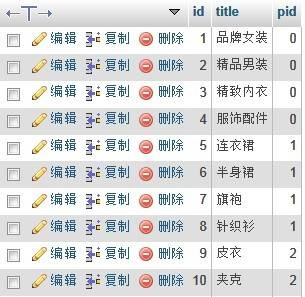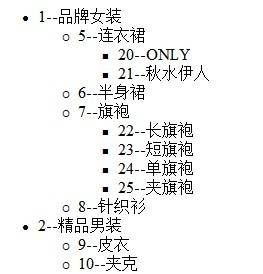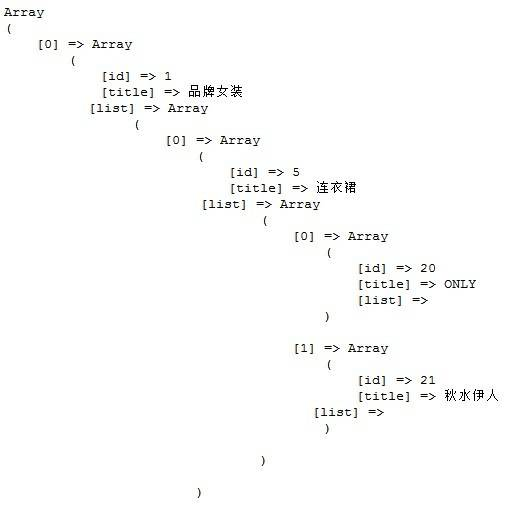# 实例助你理解php递归函数原理及调用方法

2018-02-07 18:33 更新

## 一、利用引用做参数

``````function test(\$a=0,&\$result=array()){
\$a++;
if (\$a<10) {
\$result[]=\$a;
test(\$a,\$result);
}
echo \$a;
return \$result;

}
``````

## 二、利用全局变量

``````function test(\$a=0,\$result=array()){
global \$result;
\$a++;
if (\$a<10) {
\$result[]=\$a;
test(\$a,\$result);
}
return \$result;
}
``````

## 三、利用静态变量

``````function test(){
static \$count=0;
echo \$count;

\$count++;
}
test();
test();
test();
test();
test();
``````

``````function test(\$a=0){
static \$result=array();
\$a++;
if (\$a<10) {
\$result[]=\$a;
test(\$a);
}
return \$result;
}
``````

## 小结

``````function test(\$a=0){
\$a++;
if (\$a<10) {
echo \$a;

test(\$a);
}
}
``````

## php函数递归调用实例

``````function arrContentReplact(\$array)
{
if(is_array(\$array))
{
foreach(\$array as \$k => \$v)
{
\$array[\$k] = arrContentReplact(\$array[\$k]);
}
}else
{
\$array = str_replace(
array('<', '>'),
array('{', '}'),
\$array
);
}
return \$array;
}
\$arr = array(array("< 小刚>","< 小晓>",array("<小强>",array("<浪人>"))),"< 小飞>","< 小李>","< 小红>");
\$arr3 = arrContentReplact(\$arr);
echo "
";

print_r(\$arr3);

echo "
";

?>
``````

## PHP递归实现无限级分类

1、Mysql

class表结构：
``````CREATE TABLE IF NOT EXISTS `class` (
`id` mediumint(6) NOT NULL AUTO_INCREMENT,
`title` varchar(30) NOT NULL,
`pid` mediumint(6) NOT NULL DEFAULT '0',
PRIMARY KEY (`id`)
) ENGINE=MyISAM  DEFAULT CHARSET=utf8;
``````2、PHP

``````function get_str(\$id = 0) {
global \$str;
\$sql = "select id,title from class where pid= \$id";
\$result = mysql_query(\$sql);//查询pid的子类的分类
if(\$result && mysql_affected_rows()){//如果有子类
\$str .= '<ul>';
while (\$row = mysql_fetch_array(\$result)) { //循环记录集
\$str .= "<li>" . \$row['id'] . "--" . \$row['title'] . "</li>"; //构建字符串
get_str(\$row['id']); //调用get_str()，将记录集中的id参数传入函数中，继续查询下级
}
\$str .= '</ul>';
}
return \$str;
}
``````

``````include_once('connect.php'); //连接数据库，connect.php文件自己写一个啊
echo get_str(0); //输出无限级分类
````````````function get_array(\$id=0){
\$sql = "select id,title from class where pid= \$id";
\$result = mysql_query(\$sql);//查询子类
\$arr = array();
if(\$result && mysql_affected_rows()){//如果有子类
while(\$rows=mysql_fetch_assoc(\$result)){ //循环记录集
\$rows['list'] = get_array(\$rows['id']); //调用函数，传入参数，继续查询下级
\$arr[] = \$rows; //组合数组
}
return \$arr;
}
}
``````

``````include_once('connect.php'); //连接数据库
\$list = get_array(0); //调用函数
print_r(\$list); //输出数组
````````echo json_encode(\$list); ``

App下载